# How Much Does the Earth Curve?

We’ve looked at how to find the circumference of the earth, and how far we can see over the horizon. Another kind of question we’ve had about the curvature of the earth is, how much does it curve over a given distance? That has been asked in several different ways, which lead to some intriguing insights.

## How high a bulge?

Here’s the first question, from 1997:

Earth's Curvature

I am interested in knowing the degree of curvature of the earth's surface.  I am attempting to find a method which would allow me to conceptualize and calculate the making of such a depiction on paper.

That is to say, if the earth curves from point A to point B, points exactly 10 kilometers apart, if one were to draw a straight line between the two points, then WHAT WOULD BE THE HEIGHT of the highest point the curvature AB would be above the line AB?  Moreover, if the point AB were now to be 20 kilometers apart, would the height be 2 times the height calculated earlier or would it be something altogether different?

Thank you for your kind consideration and aid.  This young artist sincerely and surely appreciates it!

Curvature is technically measured in terms of the angular turn per unit distance, in various ways; as we’ll see throughout this post, trying to measure it in terms of distances only, while it seems to be a natural idea, causes some trouble. But the second question here, about how things change when you double the distance, is just the insight we’ll need at the end.

Hi Neha -

The first thing to realize is that this problem is about circles in the plane, not about spheres in 3-dimensional space.  You are only interested in comparing a curve and a straight line, and they all lie in the same plane. A plane through a sphere yields a circle.  So we just have to understand how a circle pulls away from a straight line.

Here’s what that cross-section looks like (exaggerated as usual) as he’ll set it up in a coordinate system: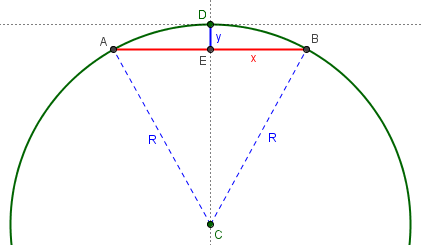Consider a circle of radius R, tangent to the x-axis at the origin and the center on the negative y-axis.  (I set it up this way in order to simplify the calculation of the height which now will go straight up the y-axis from the midpoint of line AB to the origin.) The equation for such a circle is:

x^2 + (y+R)^2 = R^2

Equivalently, x^2 + y^2 + 2Ry = 0

Equivalently, y = -(x^2 + y^2)/(2R)

He is using an unconventional trick here; note that he didn’t really solve for y, which is still on both sides of the equation, but that will disappear into an approximation:

Near the origin y is small, and y^2 is smaller still, so this can be approximated by:

y = - x^2 / (2R)

If 2 points are separated by a distance L, they might be point A at x = -L/2 and point B at x = +L/2.  At both A and B we have:

y = - L^2 / (8R)

That is, while the curve from A to B goes through the origin, the straight line from A to B cuts a distance L^2/(8R) below the origin.  Notice that doubling L (say, from 10 miles to 20) means quadrupling this distance.

Here is a picture showing the new labels: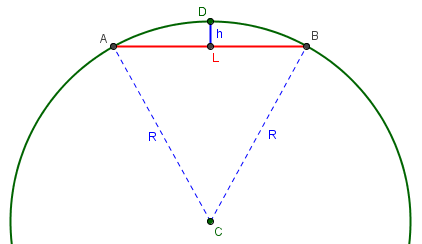When two points A and B are L units apart (“as the mole digs”, one might say), the straight line joining them is $$h \approx \frac{L^2}{8R}$$ below the surface at its midpoint. Keep in mind that this is approximate, applicable only when L (and therefore h) is much less than R.

As for the curvature of the actual earth, you just have to plug in, for R, the radius of the earth, which is about 4000 miles.  So, if L = 10 miles, then:

y = (100/32,000) miles (about 16 feet)

As we should expect, this is not a very high pile of earth, for distances at this scale; drawings such as ours above have to show huge distances to make the curve even visible.

You can see this effect in real life.  If you look across a 10-mile wide lake at the other shore, you can't see the low buildings on the other side! To see a cabin 16 feet high, you would have to climb a ladder so that your eyes are 16 feet above the ground (to be exact you would take into account the effect of the curvature on the angles of the building and your viewing station make relative to the "y-axis", but this effect is negligible at this distance).

This is dramatic in Lake Tahoe, where, from the north shore of the lake you can see the tall casinos of Stateline, Nevada, but not the low buildings of South Lake Tahoe, California.  (Lake Tahoe is also more than 10 miles from top to bottom).

Relating this bulge to the line of sight we looked at last time, we see that two points 10 miles apart would have to be 16 feet above the water level to (barely) see each other.

By the way, the L^2/(8R) answer is only an approximation, since we ignored the y^2 term in the equation of the circle, and ignored the difference between the straight-line distance between A and B and the distance along the curve.  These corrections are negligible, being approximately of size L^4/R^3. If L = 10 miles and R = 4000 miles, these corrections add up to less than 1/1000 of an inch!

We’ll see an exact formula next.

## Improving accuracy

In 2004, a student tried out the formula, not on the earth but on a drawn circle, and his question was added to that page (with wording changed accordingly):

Your reply to this question assures us that the Math is the same for all the calculations and the only things that change are the radius of the circle and the distance from a to b.

I have drawn a circle with a 60 mm radius and tried out the calculations.  Where "L" (distance between points a and b) is very short, say 40 mm, then the result "Y" is virtually accurate.

However, as you lengthen "L" to say 115 mm the result "Y" becomes wholly inaccurate.  Instead of a measured result of 42 mm the calculation result is 27 mm.

Can you shed any light on this for me please?  Am I missing something very simple?

Here is the circle with Martin’s two chords and actual measurements: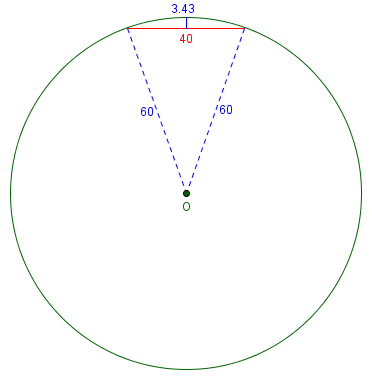$$h \approx \frac{L^2}{8R} = \frac{40^2}{8\cdot 60} = 3.33$$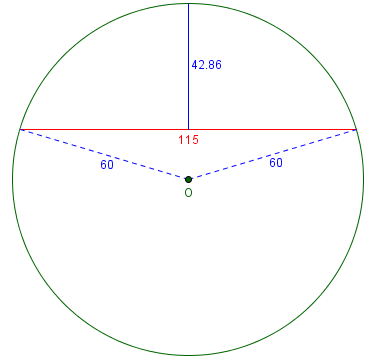$$h \approx \frac{L^2}{8R} = \frac{115^2}{8\cdot 60} = 27.55$$

Doctor Vogler replied:

Hi Martin,

Thanks for writing to Dr Math.  The last paragraph in the reply reads:

By the way, the L^2/(8R) answer is only an approximation, since we ignored the y^2 term in the equation of the circle, and ignored the difference between the straight-line distance between A and B and the distance along the curve.  These corrections are negligible, being approximately of size L^4/R^3.  If L = 10 miles and R = 4000 miles, these corrections add up to less than 1/1000 of an inch!

So essentially he is saying that when L is small compared to R, then the approximation is very good.  In your example, L is not small compared to R; in fact, L is bigger than R.

The formula is, in fact, working as advertised. And the approximation is appropriate for human distances on the earth. But what if we want to handle small circles and big chords?

So let's get a more exact result.  You'll recall that the approximation was in neglecting the y^2 term in

x^2 + y^2 + 2Ry = 0.

So what happens if we DON'T neglect the y^2 term?  Then when we substitute x = L/2, we get

y^2 + (2R)y + (L/2)^2 = 0

and we solve for y using the quadratic formula:

y = -R + (1/2)sqrt(4R^2 - L^2)

and

y = -R - (1/2)sqrt(4R^2 - L^2),

and then the height to the x axis would be

-y = R - (1/2)sqrt(4R^2 - L^2)

or

-y = R + (1/2)sqrt(4R^2 - L^2),

Note that $$-y$$ is our h; he’s made it positive.

If you look on the circle, you notice that one of these answers is measuring the height where the curved line around the circle takes the shorter way around the circle (that's the first answer) and the other gives the answer if the curved line takes the long way around the circle.

Here are the two solutions: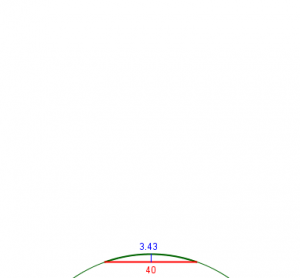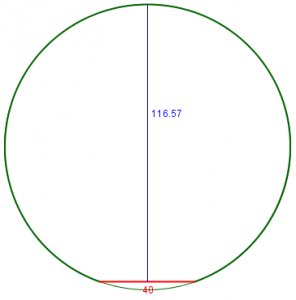The exact formula gives, respectively, $$h = R – \frac{1}{2}\sqrt{4R^2 – L^2} = 60 – \frac{1}{2}\sqrt{4\cdot 60^2 – 40^2} = 3.431$$ and $$h = R – \frac{1}{2}\sqrt{4R^2 – L^2} = 60 + \frac{1}{2}\sqrt{4\cdot 60^2 – 40^2} = 116.569$$

In the archived answer, he was looking for the short way, and you'll find that if L is small compared to R then the square root can be approximated by two terms of the Taylor series,

sqrt(4R^2 - L^2) is about 2R(1 - (1/2)(L/2R)^2)

where the difference will be roughly the size of the third term of the Taylor series, which is (1/8)(L/2R)^4.  That approximation gives the same answer as recorded in the archive, L^2/8R.  But if (L/2R)^4 is not a small number, then this is certainly not a good approximation, and so you should use the exact answer,

R - (1/2)sqrt(4R^2 - L^2).

This is an alternative way to obtain the approximation, and is also the source for the estimate of the error, “being approximately of size L^4/R^3”.

Thank you very much.  Works a treat.  Faith in math restored.

## How fast a drop-off?

A different perspective was taken by James in 2003, who referred back to that answer:

Earth's Curvature

My son and I wondered what would happen if there were no gravity.  I jokingly said that you'd take a step and simply float in a straight line forever. That got me to thinking: How far would you have to "walk" this way to get 1 foot off the ground? Scientifically, how far would you have to follow a tangent of the earth to be one foot above the surface of the curvature?

Could you use triangulation somehow?  Could you use the formula for a circle to calculate the slope?

In another discussion:

Earth's Curvature
http://mathforum.org/library/drmath/view/54904.html

you say that the formula for a circle is x^2 + (y+R)^2 = R^2 and that, "if 2 points are separated by a distance L, they might be point A at x = -L/2 and point B at x = +L/2.  At both A and B we have:

y = - L^2 / (8R)"

That leads me off in the general direction, but I'm not finishing the connection.

Doctor Jeremiah had an answer, which is closely tied to our horizon questions from last time:

Hi James,

Imagine a perfectly spherical world with a tangent:

A           B
+++++ -------+
+++    |    +++  /
+++        |        +++
+             |       /     +
+               |      /        +
+                |     /          +
+                 R   R+1           +
+                  |   /              +
+                  |  /               +
+                   | /                 +
+                   |/                  +
+                   +                   +

You travel from A to B. Your distance from the center of the Earth at A is R, and your distance from the center of the Earth at B is R+1.  This is a simple right angle triangle and can be solved for the distance from A to B with the Pythagorean formula:

(R+1)^2 = R^2 + d^2   where  d = the distance from A to B
d^2 = (R+1)^2 - R^2
d^2 = R^2+2R+1 - R^2
d^2 = 2R+1
d = sqrt(2R+1)

This is the distance to the horizon from point B, which is 1 unit above the surface.

The radius of the Earth (R) is 3963.19 statute miles or, more importantly for us, 20925643.2 feet. That means:

d = sqrt(2R+1)   where  R = 20925643.2 feet
d = sqrt(2*20925643.2+1)
d = sqrt(41851287.4)
d = 6469.26 feet

So you would have to travel along the tangent for over a mile to get one foot off the ground.

We’ll connect this to the bulge formula soon!

## 8 inches per mile?

I want to include an example of a question we got several times, that was never archived. This is from 2009:

Hi, I've read that the curvature of the earth is approximately eight inches per mile. Here's the problem. If it only curves eight inches per mile, that doesn't seem to be nearly enough to explain things we know about our planet. E.g. sailors can observe ships disappear over the horizon, but if the curvature is only eight inches per mile, then 20 miles (which is the furthest you can see a ship with the naked eye) would only give a curvature of 160 inches, or approximately 13 ft - nowhere near enough for a ship to disappear. More strikingly, at 4000 miles (approximately the radius of the planet) you would have a curvature of less than a mile. Again, that seems far too small a number. I must be missing something but I can't figure out what it is.

I'm guessing that the curvature does not merely add up in a linear fashion, e.g. if it has deviated eight inches after one mile it is not correct to assume that it has deviated sixteen inches after two miles. Perhaps the curvature grows in an exponential fashion? But I'm clutching at straws here because, frankly, I'm totally confused!

That was a pretty good guess; in fact, if you’ve been reading observantly, you will have seen a partial answer very similar to his suggestion.

That's exactly it (though not literally exponential, as we'll see). It's not appropriate to state the curvature as "8 inches PER mile", because the word "per" implies a proportionality, which is not true here. The earth presumably does curve down 8 inches in each mile, but since the direction of "down" keeps changing, they don't add up so that it goes down 8d inches in d miles. All you should say is that it goes down 8 inches in ONE mile.

Here is what I mean: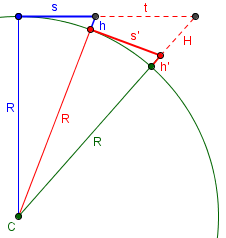If the drop of the earth over the first mile, h, is 8 inches, then if we dropped to the ground and continued another mile, we would have another drop, h‘, of 8 inches. But that mile would not be in the same direction, nor would the drop at its end. If we actually continued in the same straight line, we would have a considerably longer drop, H.

Let's look at specifics. If we were to graph a cross-section of the earth, life-size, with our location at the origin and the center below us on the y axis, the center would be at about -4000 miles, or -21,120,000 feet, or 253,440,000 inches, on the y-axis.

The equation of this circle would be

x^2 + (y + r)^2 = r^2

Solving for y,

y = sqrt(r^2 - x^2) - r

This is the drop perpendicular to our straight line, not perpendicular to the earth, which has curved away under us: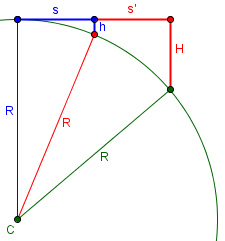Clearly, the drop is not just twice h. For the much smaller size we are discussing, the difference will not be so great, but it will still be real.

To check your claim, we can find y when x = 5280*12 = 63360 inches:

y = sqrt(253,440,000^2 - 63360^2) - 253,440,000

= -7.92 in

So the circle does indeed go down about 8 inches in a mile.

This is about what we found before, but is in a different direction this time, so it is a little more.

Let's repeat the process for 2, 3, 4, ... miles out. I'll use a spreadsheet to do it.

X             Y
63,360        -7.92 (at 1 mile)
126,720       -31.68
190,080       -71.28
253,440      -126.72
316,800      -198.00
380,160      -285.12
443,520      -388.08
506,880      -506.88
570,240      -641.52
633,600      -792.00 (at 10 miles)
6,336,000   -79,212.38 (at 100 miles)

So in 10 miles the earth drops not 80 inches, but almost 800 inches, or 66 feet -- so it's nearly quadratic (proportional to the square of the distance), which can be explained with a little more algebra. At 100 miles it's dropped 6600 feet, over a mile.

That starts to make a little more sense!

Chris responded with a couple other references:

Many thanks for a superbly comprehensive and informative answer. I really appreciate you taking the trouble. I note that I'm not the only person who was confused about the problem; if you google 'curvature earth' the first hit you get takes you to a maths discussion page not unlike Dr Math, but the answer provided makes the wrong assumption that you corrected in your answer (namely that the earth curves eight inches PER mile, therefore 80 inches for ten miles etc). A later poster corrected this misconception but it's obviously widespread! If you're interested you can find it at http://mathcentral.uregina.ca/QQ/database/QQ.09.02/shirley3.html

One of your colleagues at Dr Math does a better job but still comes up with a different figure for how much curvature there would be at 10 miles (he reckons 16 ft rather than 66 ft).

http://mathforum.org/library/drmath/view/54904.html

Anyway, either way you have done a great job of illuminating this point, and I'm very grateful for the clarity of your explanation and the amount of detail you went into. I hope you have a great weekend - as far as I'm concerned you've earned it!

Here is part of the MathCentral page:

[Question:]

There are 2 six foot men. What would the distance be between them before one could not be seen because of the curvature of the earth?

My grandson, who is stationed in Baghdad, Iraq, asked the question. This is one of the fun problems his unit was discussing. Thanks for your time.

In an earlier question on the curvature of the Earth Harley showed that the earth curves approximately 8 inches per mile. Since 6 feet is 72 inches and 9*8 = 72, the Earth curves approximately 6 feet in 9 miles. Thus the two men would have to be approximately 18 miles apart.

This answer was corrected in 2004, by finding each man’s distance to the horizon as about 3 miles, so that the correct answer is about 6 miles, not 18. If we use the approximately quadratic nature of the relationship, we might estimate that the earth will drop 9 times as much in about $$\sqrt{9} = 3$$ times as far, so, again, each man walks 3 miles and their total distance is 6 miles.

I replied first to the MathCentral error:

It's an easy thing to do if you don't think carefully!

(By the way, Ask Dr. Math has been known to make errors too. We’ve even made a couple minor errors in our current incarnation as The Math Doctors …)

Then, to the apparent discrepancy in our previous answer, which was the first answer in this post:

I think he does a great job; he filled in what I skipped over by saying "... which can be explained with a little more algebra".

As for the numerical discrepancy, he isn't dealing with the amount of dip in 10 miles, but the amount of rise BETWEEN two points 10 miles apart, which (as mentioned in the other page) is equivalent to the amount of dip in only 5 miles. My table shows a dip of 198 inches, or 16.5 feet. So he's got it just right.

It’s definitely a mind-bending problem!

This site uses Akismet to reduce spam. Learn how your comment data is processed.